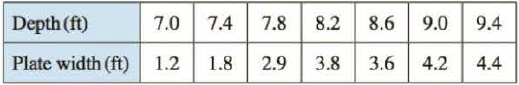Chapter 8.3, Problem 19E

Chapter
Section
Textbook Problem

A metal plate was found submerged vertically in seawater, which has density 64 lb/ft3. Measurements of the width of the plate were taken at the indicated depths. Use Simpson’s Rule to estimate the force of the water against the plate.To determine

To estimate: The force of the water against the plate using Simpson’s Rule.

Explanation

Given:

The density of water is δ=64lb/ft3.

The depth and width of the plate as shown in Table 1:

The number of subintervals as n=7.

Calculation:

The width of the plate is measured at equal interval of depth. Hence, the subinterval width (Δx) is 0.4.

Calculate the force of the water against the plate using Simpson’s Rule:

F=Δxδ3[x1w1(x1)+4x2w2(x2)+2x3w3(x3)+4x4w4(x4)+2x5w5(x5)+4x6w6(x6)+x7w7(x7)] (1)

Substitute 0.4 for Δx, 7.0 for x1, 7.4 for x2, 7.8 for x3, 8.2 for x4, 8.6 for x5, 9.0 for x6, and 9.4 for x7 in Equation (1).

F=0.4δ3[7.0w1(7.0)+4×7.4w2(7.4)+2×7.8w3(7.8)+4×8.2w4(8.2)+2×8.6w5(8.6)+4×9.0w6(9.0)+9.4w7(9.4)]=0

Still sussing out bartleby?

Check out a sample textbook solution.

See a sample solution

The Solution to Your Study Problems

Bartleby provides explanations to thousands of textbook problems written by our experts, many with advanced degrees!

Get Started

What is the slope of a nonvertical line? What can you say about the slope of a vertical line?

Applied Calculus for the Managerial, Life, and Social Sciences: A Brief Approach

In Problems 51 and 52, use a change-of-base formula to evaluate each logarithm.

Mathematical Applications for the Management, Life, and Social Sciences

The length of the curve given by x = 3t2 + 2, y = 2t3, is:

Study Guide for Stewart's Single Variable Calculus: Early Transcendentals, 8th

True or False: is a convergent series.

Study Guide for Stewart's Multivariable Calculus, 8th

Solve the equation 28A2=(5+A)(2A)for A.

Mathematics For Machine Technology